# Shapes and Angles

## Objective

Identify and draw right, obtuse, and acute angles.

## Common Core Standards

### Core Standards

?

• 4.G.A.1 — Draw points, lines, line segments, rays, angles (right, acute, obtuse), and perpendicular and parallel lines. Identify these in two-dimensional figures.

?

• 3.G.A.1

## Criteria for Success

?

1. Understand that a right angle is a square corner.
2. Understand that an acute angle is one that is less than a right angle.
3. Understand that an obtuse angle is one that is greater than a right angle but less than a straight angle.
4. Understand that a straight angle is one that is formed by three points that are all on the same line.
5. Determine whether angles are right, obtuse, or acute using a right-angle template (MP.5).
6. Draw right, obtuse, and acute angles (MP.5, MP.6).

## Tips for Teachers

?

• The following materials are needed for today's lesson: Blank Paper
• Note that students will later see that a right angle is the angle formed by perpendicular lines and measures 90°, more precise definitions of right angles. However, because these terms and concepts have not yet been introduced, the definition is somewhat informal for the time being.
• Students are going to create a right-angle template in this lesson. Have students save this template for future lessons.

#### Remote Learning Guidance

If you need to adapt or shorten this lesson for remote learning, we suggest prioritizing Anchor Task 2 (benefits from worked example). Find more guidance on adapting our math curriculum for remote learning here.

#### Fishtank Plus

• Problem Set
• Student Handout Editor
• Vocabulary Package

?

### Problem 1

Sort the shapes (cut out from Template: Shapes) into groups. You may sort them any way you want and into as many groups as you want.

#### References

EngageNY Mathematics Grade 4 Mathematics > Module 4 > Topic A > Lesson 2Concept Development Problem 1

Grade 4 Mathematics > Module 4 > Topic A > Lesson 2 of the New York State Common Core Mathematics Curriculum from EngageNY and Great Minds. © 2015 Great Minds. Licensed by EngageNY of the New York State Education Department under the CC BY-NC-SA 3.0 US license. Accessed Dec. 2, 2016, 5:15 p.m..

Modified by The Match Foundation, Inc.

### Problem 2

Determine whether each of the angles (on Template: Angles) are less than, greater than, or equal to right angles.

#### References

EngageNY Mathematics Grade 4 Mathematics > Module 4 > Topic A > Lesson 2Concept Development Problem 2

Grade 4 Mathematics > Module 4 > Topic A > Lesson 2 of the New York State Common Core Mathematics Curriculum from EngageNY and Great Minds. © 2015 Great Minds. Licensed by EngageNY of the New York State Education Department under the CC BY-NC-SA 3.0 US license. Accessed Dec. 2, 2016, 5:15 p.m..

Modified by The Match Foundation, Inc.

### Problem 3

Draw an example of a right angle, an acute angle, an obtuse angle, and a straight angle.

#### References

EngageNY Mathematics Grade 4 Mathematics > Module 4 > Topic A > Lesson 2Concept Development Problem 3

Grade 4 Mathematics > Module 4 > Topic A > Lesson 2 of the New York State Common Core Mathematics Curriculum from EngageNY and Great Minds. © 2015 Great Minds. Licensed by EngageNY of the New York State Education Department under the CC BY-NC-SA 3.0 US license. Accessed Dec. 2, 2016, 5:15 p.m..

## Problem Set & Homework

#### Discussion of Problem Set

• #1(c) and 1(f) are both right angles. Describe their position. Does the orientation of an angle determine whether it is right, acute, or obtuse?
• In #3(a), each ray shared the same endpoint. The shared endpoint is called a vertex. Label the points on your angles in Problem 3. Identify the vertex in Problems 3(b) and 3(c) with your partner.
• What is the difference between a straight angle and a line?
• Where else in your environment have you seen right angles?
• How did the right-angle template help you recognize and draw angles? How can a right-angle template help you recognize an acute angle?
• How does the right-angle template help you visualize the interior of an angle? Where would I find the interior of an angle that I’ve drawn?
• What does the exterior of an angle refer to?

?

### Problem 1

Look at the following angles.

A                   B                   C               D                  E                      F                  G                H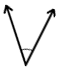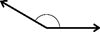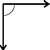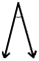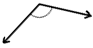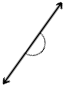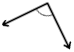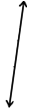1. Which angles are right angles?
2. Which angles are obtuse angles?
3. Which angles are acute angles?
4. Which angles are straight angles?

#### References

EngageNY Mathematics Grade 4 Mathematics > Module 4 > Topic A > Lesson 2Exit Ticket, Question #2

Grade 4 Mathematics > Module 4 > Topic A > Lesson 2 of the New York State Common Core Mathematics Curriculum from EngageNY and Great Minds. © 2015 Great Minds. Licensed by EngageNY of the New York State Education Department under the CC BY-NC-SA 3.0 US license. Accessed Dec. 2, 2016, 5:15 p.m..

Modified by The Match Foundation, Inc.

### Problem 2

Draw an additional example of each type of angle mentioned above.

1. Right angle:
2. Obtuse angle:
3. Acute angle:
4. Straight angle:

### Mastery Response

?